TL;DR

### 环境

IDA Pro + Jadx + 耐心

# 初步分(懵)析(逼)

```package com.q2zy.babyandroid;

public class MainActivity extends AppCompatActivity implements OnClickListener {
private static String TAG = "java";

public static native boolean check(String str);

public static native boolean check0(String str);

public static native boolean check1(String str);

public static native boolean check2(String str);

public static native boolean check3(String str);

public static native boolean check4(String str);

public static native boolean check5(String str);

public static native String stringFromJNI();

static {
}

protected void onCreate(Bundle savedInstanceState) {
super.onCreate(savedInstanceState);
setContentView((int) R.layout.activity_main);
Log.w(TAG, "oncreate");
}

public void onClick(View v) {
EditText editText = (EditText) findViewById(R.id.editText);
TextView tv = (TextView) findViewById(R.id.sample_text);
String input = null;
if (editText != null) {
input = editText.getText().toString();
}
if (tv != null) {
tv.setText(check0(input) ? "flag is your input" : "failed");
}
}

public void xxx() {
Log.w(TAG, "enter xxx");
try {
} catch (Exception e) {
Log.w(TAG, e.getClass().getName());
Log.w(TAG, e);
}
Log.w(TAG, "exit xxx");
}

public static void load(String libName) {
try {
Log.w(TAG, "log b in java");
Runtime runtime = Runtime.getRuntime();
method.setAccessible(true);
} catch (Exception e) {
Log.w(TAG, e);
}
}

public static void loadLibrary(String s) {
}
}
```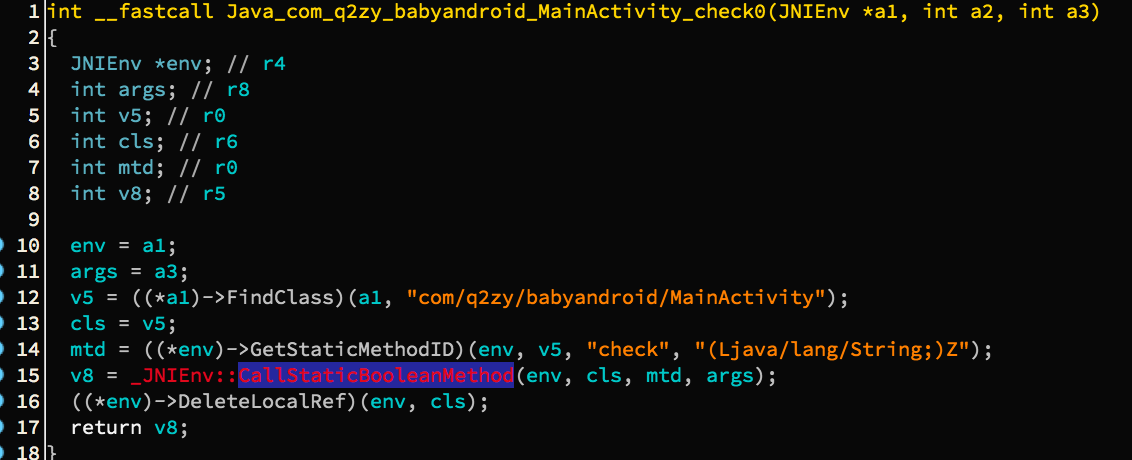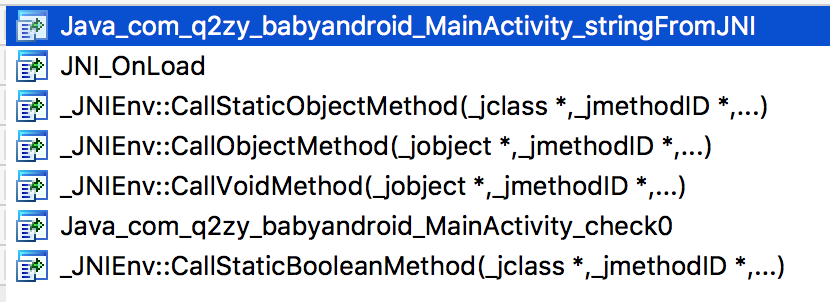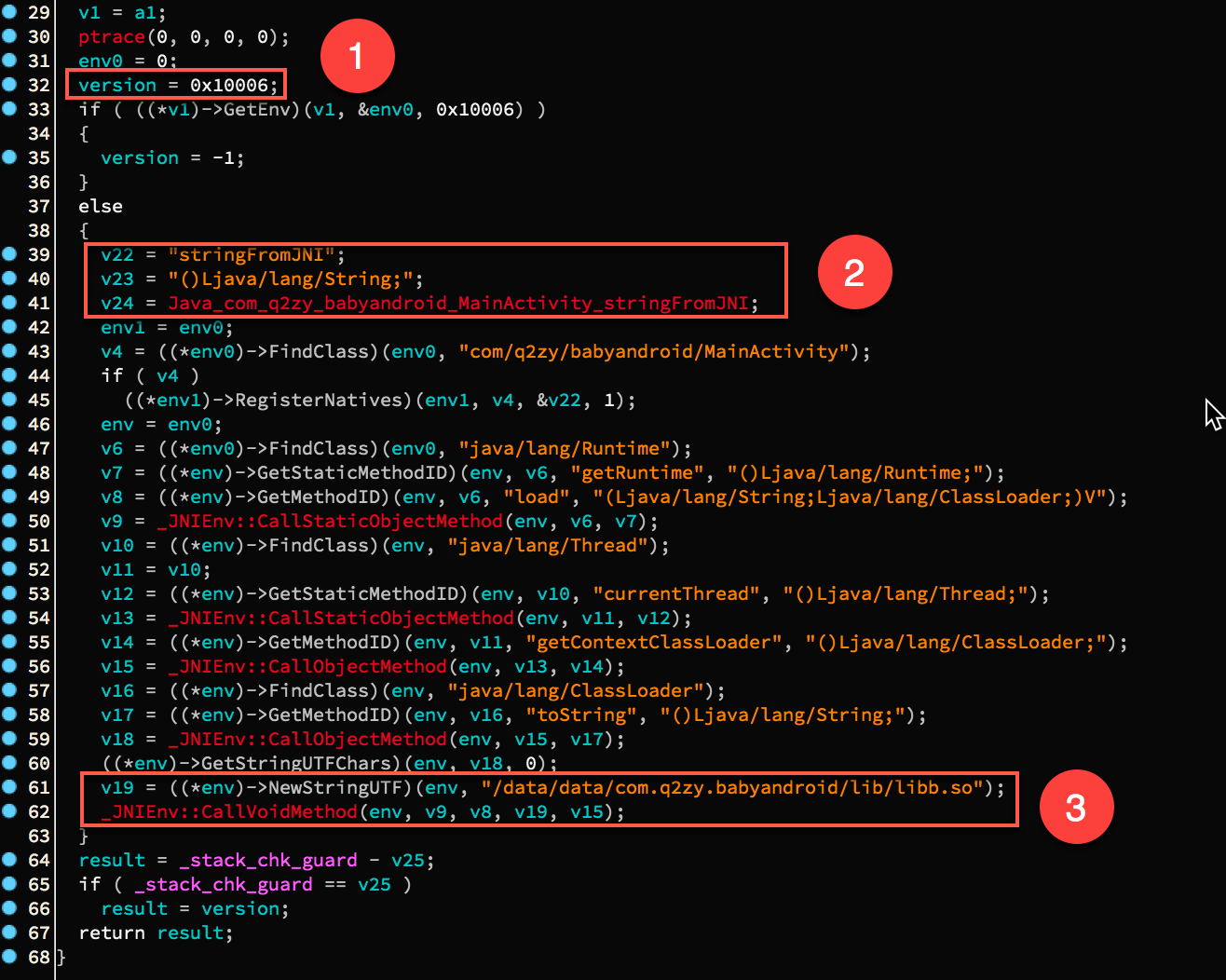1 处是 JNI 的版本,这是个常量. 然后是 2 中注册了一个 stringFromJNI 方法.

3 处是调用 java 层的 load 函数,对 libb.so 进行加载. 于是用 IDA 打开 libb,会提示 SHT invalid,不用管.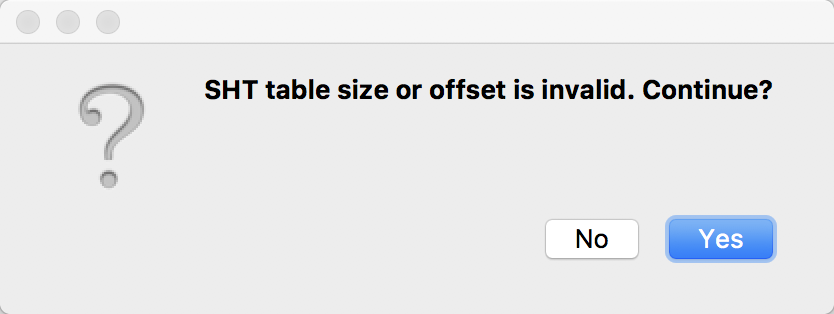里面只有一个能看到的到处函数 JNI_OnLoad 函数. 那就只能分析这个函数看看做了什么. 然后欣喜的打开 JNI_OnLoad, 结果发现就长这鬼样子.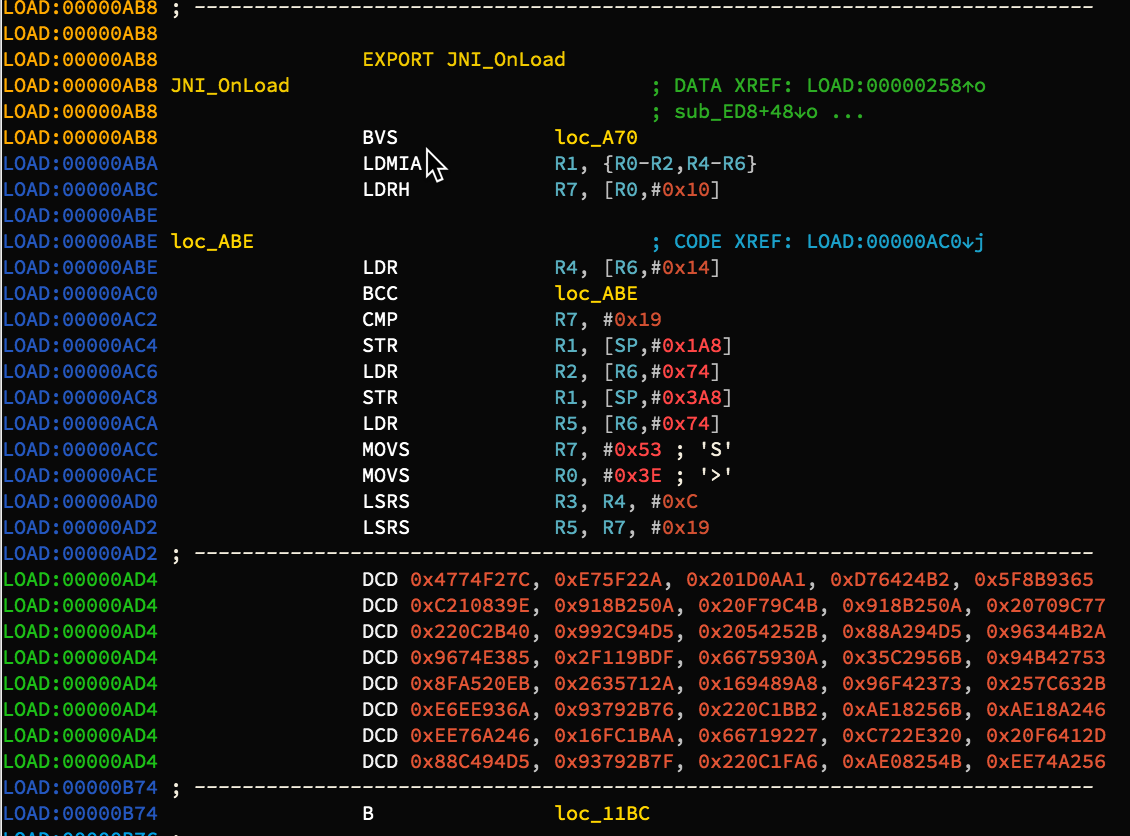# 解密 libb.so 的 JNI_OnLoad 函数

```off_xxx 表示偏移为 xxx 处的值

0x4008 处的值是 0x4C11DB7,典型的 crc32 生成多项式
```

```// liba.so JNI_OnLoad 方法的前四个字节
unsigned encry = 0xc977d6da;
// 得到解密秘钥
unsigned key = jniload_sig ^ encry;
printf("%#x\n", key);
// 输出得到 key = 0x6674632a
```

```
unsigned* getkey(const char *a1)
{
FILE *v1; // r6
signed int crc; // r4
int *pgen1; // r5
int *pgen2; // r11
size_t end; // r10
int i; // r6
int j; // r9
unsigned int abyte; // r8
int v9; // r0
int v10; // r0
int v11; // r1
unsigned *result; // r0
FILE *stream; // [sp+0h] [bp-8028h]
char data; // [sp+4h] [bp-8024h]
int v15; // [sp+8004h] [bp-24h]

v1 = fopen(a1, "rb");
rewind(v1);
crc = -1;
stream = v1;
int gen = 0x4C11DB7;
pgen1 = &gen;
pgen2 = &gen;
// printf("condition = %x\n",(*pgen1) & 1);
do
{
end = fread(data, 1u, 0x8000u, v1);
if ( end )
{
i = 0;
do
{
j = 0;
abyte = data[i];
do
{
v9 = crc ^ (abyte >> j << 31);
crc *= 2;
if ( v9 <= -1 )
{
v10 = *pgen1;
crc ^= v10;
}
++j;
}
while ( j != 8 );
++i;
}
while ( i != end );
}
v1 = stream;
}
while ( !feof(stream) );
fclose(stream);
result = calloc(5u, 1u);
*result = ~crc;
return result;
}

```

```
FILE* fso = fopen("./libb.so", "rb");
char so[0x40b0];
memset(so, 0, 0x40b0);

int cnt = fread(so, 1, 0x40b0, fso);
#ifdef DEBUG
for(int i = 0;i < 0x10; ++i) {
printf("%x\n",so[i]);
}
printf("\n");
#endif

unsigned* encry = (unsigned*)(so + 0xab8);
printf("First four bytes: %#x\n", *encry);
for(unsigned* k = encry; k < encry + 0x420; ++k) {
/*printf("BEFORE: %x\n", *k);*/
*k ^= key;
/*printf("AFTER: %x\n", *k);*/
}
fclose(fso);
fwrite(so, 1, 0x40b0, fwso);
fclose(fwso);
}

```

```
int main() {
const char* fname = "./libb.so";
unsigned *key = getkey(fname);
printf("Check sum is %#x\n", *key);
return 0;
}

```

```int __cdecl _aeabi_memcpy(void *, const void *, size_t)
```

```
loopkey = initkey
while True:
cnt += 1
b = loopkey ^ key
print('[+] %s => %s' % (hex(bb), hex(idc.Byte(addr - 1))))
print("[*] Decrypt and patch %d bytes from %s ." % (cnt, hex(savedaddr)))

```

```decrypt(0x2010,0xf0,0x93); decrypt(0xcb4,0x73,0x10); decrypt(0x2038,0x3e,0x16)
```

```
def decrypt_tail():
index, end = 0x27c, 0x420
base = 0xAB9 & 0xFFFFFFFE
key = 0x6674632a
while True:
index += 4
if(index >= end):break

```

# 最后的战役

```
def decrypt_final():

# 1: 获取加密后的字节数组
secret = []
secret_base = 0xe54
while True:
b = idc.Byte(secret_base)
if(not b):break
secret.append(hex(b)[2:].zfill(2))
secret_base += 1

# 2: 从 list 中索引 index 处获得一个整数
def getint_fromlist(index, lst):
end = len(lst) - 1 if index + 3 > len(lst) - 1 else index + 3
num = ""
for i in range(end, index - 1, -1):
hx = lst[i]
num += hx
return int(num,16)

# 3: 在 list 索引 index 处放入一个整数 num
def setint_tolist(index, lst, num):
num = hex(num)[2:].zfill(8)
numlst = re.findall(r".{1,2}", num)
lsb = numlst[-1::-1]
end = len(lst) - 1 if index + 3 > len(lst) - 1 else index + 3
lst[index:end + 1] = lsb[0:end + 1 - index]

# 4: 模拟加密(a)
key = 0x6674632a
square_key = key * key

v = getint_fromlist(19, secret)
setint_tolist(19, secret, (square_key >> 32) ^ v)
vv = getint_fromlist(15, secret)
setint_tolist(15, secret, vv ^ square_key)

# 5: 模拟加密(b)
for i in range(8):
v = getint_fromlist(i, secret)
num = getint_fromlist(i + 4, secret)
setint_tolist(i, secret, v ^ square_key)
setint_tolist(i + 4, secret, num ^ (square_key >> 32))

# 6: 打印 flag
flag = [chr(int(c,16)) for c in secret]
print("[+] flag is %s" % (''.join(flag)))

```

#1 节用于获取加密后的字节数组, 其基地址是 0x54,存放时要保证存放每个字符的十六进 制形式,不包括前缀 0x,且长度为2. 比如一个字节为 0xf,则应该去掉 '0x' 并补 0 得到 "0f" 放入列表中,该列表诸如 lst = ["01","00","0a","1b","2c"] 等形式, 以实际内存存储字节的方式来存储字节序列.

#2 和 #3 主要用于从列表中获取一个整数,由于是小段序列,所以我们获取整数时, 需要从后往前读取. 比如说从 lst 中获取索引 0 处的整数,得到的是 0x1b0a0001. 则 getint_fromlist(0,lst) 返回一个整数 0x1b0a0001. 而setint_tolist 恰好相反, 对输入的数值,先转换为十六进制字符串, 然后 0 填充至长度为 8,写入 lst 的时候要按照小段序列填充.

#4 和 #5 就是按照加密顺序在反向加密一下,这个对照 IDA 的反编译代码和 #2,#3 处的辅助函数, 不是很难理解.

#5 处就是打印输出 flag.

```Python>decrypt_final()
[+] flag is 1nTr3stIng-AnDr0id-A&D!
```

# P.S.

Any question? 欢迎联系我,交朋友或者探讨问题,都 OK!

# 代码下载

C 代码 && idapython 脚本函数

# Reference

1. Understanding and implementing CRC (Cyclic Redundancy Check) calculation

Contact me by dXAyZ2Vla0AxNjMuY29tCg==
OR